# Congruence and congruent triangles

### Congruence and congruent triangles

#### Lessons

• Introduction
a)
1. Similar tirangles VS. Congruent triangles
2. Ways to prove congruency:
• SSS
• SAS
• ASA
• AAS

• 1.
Which pairs of triangles are congruent?
a)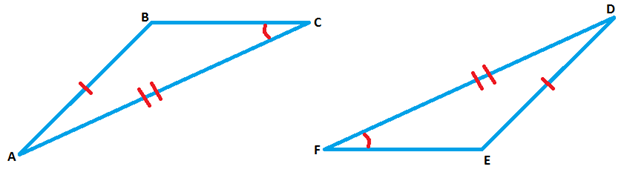b)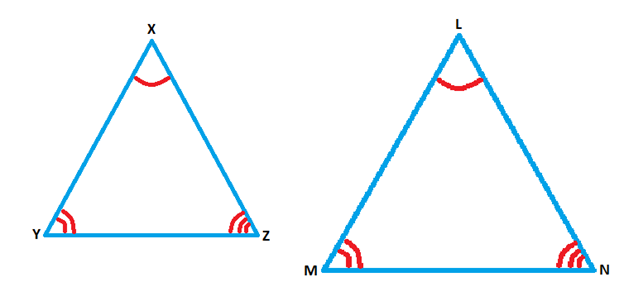c)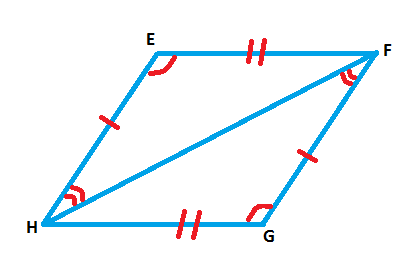• 2.
In the following diagram, $\triangle\ GHK$ $\cong$ $\triangle\ QRS$.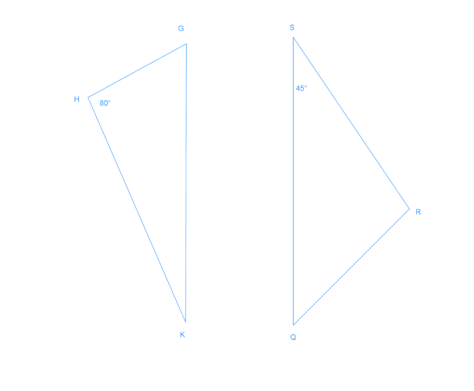i) Find.
a)
m$\angle\ G$ = ?

b)
m$\angle\ R$ = ?

c)
SR = ?

d)
$\overline{HK}$ = ?

e)
Find m$\angle\ R$.

• 3.
The gardener wants to divide a rectangular flower bed into 2 parts as shown in the following figure. Identify if the two parts are the same size and shape.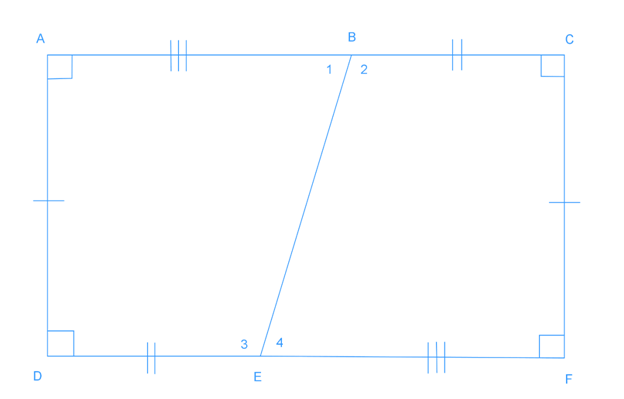• 4.
Write a two-column proof.
a)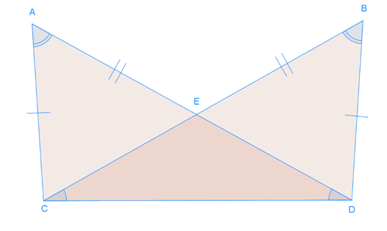Given: $\overline{AC}$$\cong$$\overline{BD}$, $\overline{AD}$$\cong$$\overline{BC}$, $\angle\ CAD$$\cong$$\angle\ CBD$, $\angle\ ADC$$\cong$$\angle\ BCD$
Prove: $\triangle\ ACD$$\cong$$\triangle\ BDC$

b)
Given: $\overline{XC}$$\cong$$\overline{ZC}$
$\overline{CY}$ bisects $\overline{XZ}$
Prove: $\triangle\ XCY$$\cong$$\triangle\ ZCY$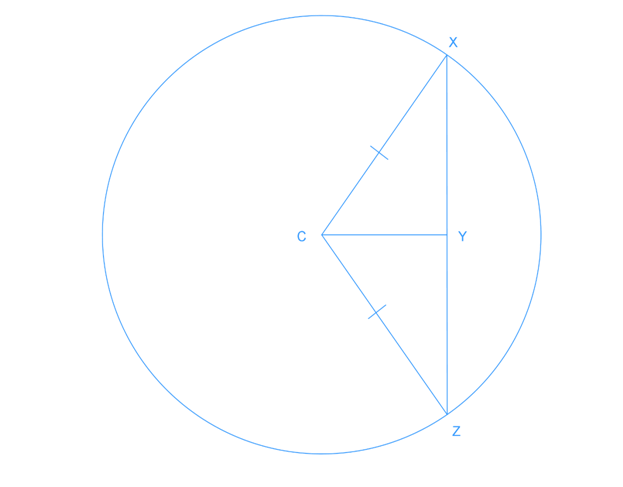• 5.
Write a flow proof
a)
Given: $\overline{AC}$$\cong$$\overline{BD}$, $\overline{AD}$$\cong$$\overline{BC}$, $\angle\ CAD$$\cong$$\angle\ CBD$, $\angle\ ADC$$\cong$$\angle\ BCD$
Prove: $\triangle\ ACD$$\cong$$\triangle\ BDC$b)
Given: $\overline{XC}$$\cong$$\overline{ZC}$
$\overline{CY}$ bisects $\overline{XZ}$
Prove: $\triangle\ XCY$$\cong$$\triangle\ ZCY$